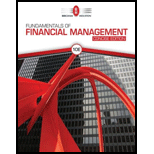# EVALUATING RISK AND RETURN Stock X has a 10% expected return, a beta coefficient of 0.9, and a 35% standard deviation of expected returns. Stock Y has a 12.5% expected return, a beta coefficient of 1.2, and a 25% standard deviation. The risk-free rate is 6%, and the market risk premium is 5%. a. Calculate each stock’s coefficient of variation. b. Which stock is riskier for a diversified investor? c. Calculate each stock’s required rate of return. d. On the basis of the two stocks’ expected and required returns, which stock would be more attractive to a diversified investor? e. Calculate the required return of a portfolio that has $7,500 invested in Stock X and$2,500 invested in Stock Y. f. If the market risk premium increased to 6%, which of the two stocks would have the larger increase in its required return?### Fundamentals Of Financial Manageme...

10th Edition
Eugene F. Brigham + 1 other
Publisher: Cengage Learning
ISBN: 9781337902571

#### Solutions

Chapter
Section### Fundamentals Of Financial Manageme...

10th Edition
Eugene F. Brigham + 1 other
Publisher: Cengage Learning
ISBN: 9781337902571
Chapter 8, Problem 19P
Textbook Problem
186 views

## EVALUATING RISK AND RETURN Stock X has a 10% expected return, a beta coefficient of 0.9, and a 35% standard deviation of expected returns. Stock Y has a 12.5% expected return, a beta coefficient of 1.2, and a 25% standard deviation. The risk-free rate is 6%, and the market risk premium is 5%. a. Calculate each stock’s coefficient of variation. b. Which stock is riskier for a diversified investor? c. Calculate each stock’s required rate of return. d. On the basis of the two stocks’ expected and required returns, which stock would be more attractive to a diversified investor? e. Calculate the required return of a portfolio that has $7,500 invested in Stock X and$2,500 invested in Stock Y. f. If the market risk premium increased to 6%, which of the two stocks would have the larger increase in its required return?

a.

Summary Introduction

To determine: The coefficient of variation for each stock.

Portfolio:

The portfolio refers to a group of financial assets like bonds, stocks, and equivalents of cash. The portfolio is held by investors and financial users. A portfolio beta is constructed in accordance with the risk tolerance and the objectives of the company.

The Coefficient of Variation:

The coefficient of variation is a tool to determine the risk. It determines the risk per unit of return. It is used for measurement of data’s with the same expected return.

### Explanation of Solution

Given,

For stock X,

The expected return is 10%.

The beta coefficient is 0.9.

The standard deviation is 35%.

For stock Y,

The expected return is 12.5%.

The beta coefficient is 1.2.

The standard deviation is 25%.

The risk-free rate is 6%.

The market risk premium is 5%.

The formula to calculate the coefficient of variation is,

Coefficientofvariation=Standarddeviation(σ)Expectedreturn(r)

Calculation of coefficient of variation for Stock X,

Substitute 35% for standard deviation and 10% for expected return on stock in the above formula

b.

Summary Introduction

To determine: The riskier stock for a diversified investor.

c.

Summary Introduction

To determine: The required rate of return for each stock.

The Required Rate of Return:

The required rate of return is the rate which should be earned on an investment to keep that investment running in the market. When the required return is earned only then the users and the companies invest in that particular investment.

d.

Summary Introduction

To determine: The more attractive stock for a diversified investor.

e.

Summary Introduction

To determine: The required rate of return on a portfolio.

f.

Summary Introduction

To determine: The stock which has a larger increase in required return when market risk premium is increased.

### Still sussing out bartleby?

Check out a sample textbook solution.

See a sample solution

#### The Solution to Your Study Problems

Bartleby provides explanations to thousands of textbook problems written by our experts, many with advanced degrees!

Get Started

Find more solutions based on key concepts
What are the advantages of a partnership?

Foundations of Business (MindTap Course List)

What is a three-way match?

Accounting Information Systems

What is cycle time? Velocity?

Managerial Accounting: The Cornerstone of Business Decision-Making

NPV PROFILES: TIMING DIFFERENCES An oil-drilling company must choose between two mutually exclusive extraction ...

Fundamentals of Financial Management, Concise Edition (with Thomson ONE - Business School Edition, 1 term (6 months) Printed Access Card) (MindTap Course List)